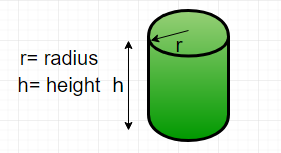# Python Program for Find the perimeter of a cylinder

• Last Updated : 06 Feb, 2018

Given diameter and height, find the perimeter of a cylinder.

Perimeter is the length of the outline of a two – dimensional shape. A cylinder is a three – dimensional shape. So, technically we cannot find the perimeter of a cylinder but we can find the perimeter of the cross-section of the cylinder. This can be done by creating the projection on its base, thus, creating the projection on its side, then the shape would be reduced to a rectangle.Formula :
Perimeter of cylinder ( P ) =here d is the diameter of the cylinder
h is the height of the cylinder

Examples :

```Input : diameter = 5, height = 10
Output : Perimeter = 30

Input : diameter = 50, height = 150
Output : Perimeter = 400
```

## Python

 `# Function to calculate ``# the perimeter of a cylinder``def` `perimeter( diameter, height ) :``    ``return` `2` `*` `( diameter ``+` `height ) `` ` `# Driver function``diameter ``=` `5` `;``height ``=` `10` `;``print` `(``"Perimeter = "``,``            ``perimeter(diameter, height))`

Output :

```Perimeter = 30 units
```

Please refer complete article on Find the perimeter of a cylinder for more details!

My Personal Notes arrow_drop_up